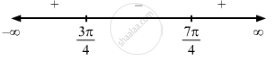Share

F(X) = Sin X − Cos X, 0 < X<2 π . - CBSE (Arts) Class 12 - Mathematics

Question

f(x) =  sin x $-$ cos x, 0 < x < 2$\pi$ .

Solution

$\text { Given: } \hspace{0.167em} f\left( x \right) = \sin x - \cos x$

$\Rightarrow f'\left( x \right) = \cos x + \sin x$

$\text { For a local maximum or a local minimum, we must have }$

$f'\left( x \right) = 0$

$\Rightarrow \cos x + \sin x = 0$

$\Rightarrow \cos x = - \sin x$

$\Rightarrow \tan x = - 1$

$\Rightarrow x = \frac{3\pi}{4} or \frac{7\pi}{4}$Sincef '(x) changes from positive to negative when x increases through $\frac{3\pi}{4}$, x = $\frac{3\pi}{4}$ is the point of local maxima.

The local maximum value of  f (x)  at x = $\frac{3\pi}{4}$ is given by $\sin\left( \frac{3\pi}{4} \right) - \cos\left(\frac{3\pi}{4} \right) = \sqrt{2}$

Since f '(x) changes from negative to positive when x increases through $\frac{7\pi}{4}$,x= $\frac{7\pi}{4}$ is the point of local minima.

The local minimum value of  f (x)  at x = $\frac{7\pi}{4}$  is given by $\sin\left( \frac{7\pi}{4} \right) - \cos\left(\frac{7\pi}{4} \right) = - \sqrt{2}$

Is there an error in this question or solution?

Video TutorialsVIEW ALL 

Solution F(X) = Sin X − Cos X, 0 < X<2 π . Concept: Graph of Maxima and Minima.
S